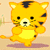Discussion about math, puzzles, games and fun.   Useful symbols: ÷ × ½ √ ∞ ≠ ≤ ≥ ≈ ⇒ ± ∈ Δ θ ∴ ∑ ∫  π  -¹ ² ³ °

You are not logged in.

## #1 2005-03-06 22:33:48

Ty
Guest

### generalizations help!

when you mulitply an even by an odd number, you always get an even number, right?

but how could you show this is algebra?

any help is much appreciated

thanks

## #2 2005-03-06 23:47:36

MathsIsFunRegistered: 2005-01-21
Posts: 7,687

### Re: generalizations help!

I may have to change my answer after thinking, but here is my "gut feel":

An even number is, by definition, able to be divided evenly by 2. So any whole number multiple of an even number (the multiple could be odd or even) must also be able to be divided evenly by 2.

In algebra it may go something like this:

A number "x" is even if x=2n, where n is an integer

And a number "y" is odd if y=2m+1, where m is an integer

So, multiplying any "x" and "y" gives: xy=2n(2m+1)

But we know the definition of even is 2 times an integer ...

So, if n(2m+1) is an integer (we may need to prove that), then 2n(2m+1) will be even, and xy must be even.

"The physicists defer only to mathematicians, and the mathematicians defer only to God ..."  - Leon M. Lederman

Offline

## #3 2005-03-07 12:59:07

LASER4
Member
Registered: 2005-03-07
Posts: 1

### Re: generalizations help!

I would like to know the digit and the word of numbers and how far do we get in naming the numbers. Lastly when in this chart would we then just use powers of numbers? Is there a point when powers are sequenced?.
For example      5 = five
50 = fifty
100 = one hundred
1 000 = one thousand
100 000 = one hundred thousand

As far as I know we get to a trillion what continues on from there. I have look in many places for such a chart to describe this but have been unable to find one.  Thanks for that

Offline

## #4 2005-03-07 15:46:41

MathsIsFunRegistered: 2005-01-21
Posts: 7,687

### Re: generalizations help!

We have up to a septillion here

You can use powers of 10 any time, really. Scientists love them, even for small numbers like 1.2 x 10^3 instead of 1200.

"The physicists defer only to mathematicians, and the mathematicians defer only to God ..."  - Leon M. Lederman

Offline

## #5 2005-03-07 18:26:26

Titus
MemberRegistered: 2005-03-07
Posts: 10

### Re: generalizations help!

MathsIsFun wrote:

I may have to change my answer after thinking, but here is my "gut feel":

An even number is, by definition, able to be divided evenly by 2. So any whole number multiple of an even number (the multiple could be odd or even) must also be able to be divided evenly by 2.

In algebra it may go something like this:

A number "x" is even if x=2n, where n is an integer

And a number "y" is odd if y=2m+1, where m is an integer

So, multiplying any "x" and "y" gives: xy=2n(2m+1)

But we know the definition of even is 2 times an integer ...

So, if n(2m+1) is an integer (we may need to prove that), then 2n(2m+1) will be even, and xy must be even.

thanks so much for that!
just one more thing on that, do we just assume that 2n(2m+1) is even, or is this because by using the 2 before the brakctes, we are saying everything is mulitplied by 2 and therefor even?

Last edited by Titus (2005-03-07 18:32:14)

Offline

## #6 2005-03-07 21:40:16

MathsIsFunRegistered: 2005-01-21
Posts: 7,687

### Re: generalizations help!

Yes, 2 times an integer is even, by definition.

And you could prove that n(2m+1) is in integer by just going through the motions of proving that 2m is an integer, +1 is still an integer, etc. Anyway, it "stands to reason" that n(2m+1) is an integer, that'll do me, cuz I aint that strict!!

"The physicists defer only to mathematicians, and the mathematicians defer only to God ..."  - Leon M. Lederman

Offline